Next: Calculation of Specific Heats Up: Classical Thermodynamics Previous: Ideal Gas Equation of

# Specific Heat

Suppose that a body absorbs an amount of heat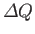, and its temperature consequently rises by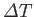. The usual definition of the heat capacity, or specific heat, of the body is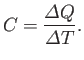(6.26)

If the body consists ofmoles of some substance then the molar specific heat (i.e., the specific heat of one mole of this substance) is defined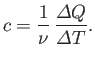(6.27)

In writing the previous expressions, we have tacitly assumed that the specific heat of a body is independent of its temperature. In general, this is not true. We can overcome this problem by only allowing the body in question to absorb a very small amount of heat, so that its temperature only rises slightly, and its specific heat remains approximately constant. In the limit that the amount of absorbed heat becomes infinitesimal, we obtain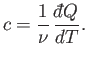(6.28)

In classical thermodynamics, it is usual to define two molar specific heats. Firstly, the molar specific heat at constant volume, denoted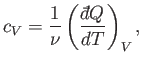(6.29)

and, secondly, the molar specific heat at constant pressure, denoted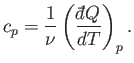(6.30)

Consider the molar specific heat at constant volume of an ideal gas. Because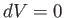, no work is done by the gas on its surroundings, and the first law of thermodynamics reduces to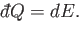(6.31)

It follows from Equation (6.29) that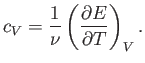(6.32)

Now, for an ideal gas, the internal energy is volume independent. [See Equation (6.25).] Thus, the previous expression implies that the specific heat at constant volume is also volume independent. Because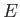is a function ofonly, we can write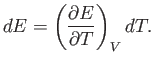(6.33)

The previous two expressions can be combined to give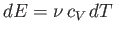(6.34)

for an ideal gas.

Let us now consider the molar specific heat at constant pressure of an ideal gas. In general, if the pressure is kept constant then the volume changes, and so the gas does work on its environment. According to the first law of thermodynamics,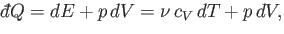(6.35)

where use has been made of Equation (6.34). The equation of state of an ideal gas, (6.10), implies that if the volume changes by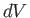, the temperature changes by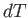, and the pressure remains constant, then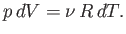(6.36)

The previous two equations can be combined to give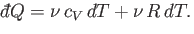(6.37)

Now, by definition,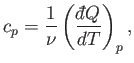(6.38)

so we obtain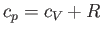(6.39)

for an ideal gas. Note that, at constant volume, all of the heat absorbed by the gas goes into increasing its internal energy, and, hence, its temperature, whereas, at constant pressure, some of the absorbed heat is used to do work on the environment as the volume increases. This means that, in the latter case, less heat is available to increase the temperature of the gas. Thus, we expect the specific heat at constant pressure to exceed that at constant volume, as indicated by the previous formula.

The ratio of the two specific heats,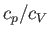, is conventionally denoted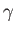. We have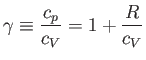(6.40)

for an ideal gas. In fact,is easy to measure experimentally because the speed of sound in an ideal gas takes the form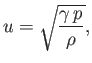(6.41)

where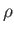is the mass density. (See Exercise 8.) Table 6.1 lists some experimental measurements of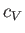andfor common gases. The extent of the agreement betweencalculated from Equation (6.40) and the experimentalis remarkable.

Table 6.1: Molar specific heats of common gases in joules/mole/degree (at 15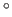C and 1 atmosphere). From Reif.Gas Symbol (experiment) (experiment) (theory) Helium He 12.5 1.666 1.666 Argon Ar 12.5 1.666 1.666 Nitrogen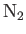20.6 1.405 1.407 Oxygen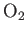21.1 1.396 1.397 Carbon Dioxide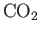28.2 1.302 1.298 Ethane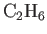39.3 1.220 1.214Next: Calculation of Specific Heats Up: Classical Thermodynamics Previous: Ideal Gas Equation of
Richard Fitzpatrick 2016-01-25